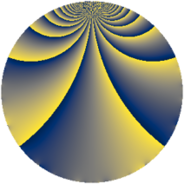# Properties

 Label 1045.2.pLevel $1045$ Weight $2$ Character orbit 1045.p Rep. character $\chi_{1045}(791,\cdot)$ Character field $\Q(\zeta_{6})$ Dimension $160$ Sturm bound $240$

# Related objects

## Defining parameters

 Level: $$N$$ $$=$$ $$1045 = 5 \cdot 11 \cdot 19$$ Weight: $$k$$ $$=$$ $$2$$ Character orbit: $$[\chi]$$ $$=$$ 1045.p (of order $$6$$ and degree $$2$$) Character conductor: $$\operatorname{cond}(\chi)$$ $$=$$ $$209$$ Character field: $$\Q(\zeta_{6})$$ Sturm bound: $$240$$

## Dimensions

The following table gives the dimensions of various subspaces of $$M_{2}(1045, [\chi])$$.

Total New Old
Modular forms 248 160 88
Cusp forms 232 160 72
Eisenstein series 16 0 16

## Trace form

 $$160 q + 12 q^{3} - 80 q^{4} + 92 q^{9} + O(q^{10})$$ $$160 q + 12 q^{3} - 80 q^{4} + 92 q^{9} + 8 q^{11} - 12 q^{14} - 80 q^{16} + 30 q^{22} - 8 q^{23} - 80 q^{25} - 8 q^{26} - 36 q^{34} + 112 q^{36} - 24 q^{38} - 72 q^{42} - 36 q^{44} + 16 q^{47} - 48 q^{48} - 136 q^{49} - 72 q^{53} + 104 q^{58} - 72 q^{59} + 208 q^{64} - 26 q^{66} - 36 q^{67} - 72 q^{70} - 12 q^{71} - 60 q^{77} + 60 q^{78} - 16 q^{80} - 72 q^{81} - 60 q^{82} + 12 q^{86} + 36 q^{89} + 24 q^{91} + 16 q^{92} + 132 q^{97} + 52 q^{99} + O(q^{100})$$

## Decomposition of $$S_{2}^{\mathrm{new}}(1045, [\chi])$$ into newform subspaces

The newforms in this space have not yet been added to the LMFDB.

## Decomposition of $$S_{2}^{\mathrm{old}}(1045, [\chi])$$ into lower level spaces

$$S_{2}^{\mathrm{old}}(1045, [\chi]) \cong$$ $$S_{2}^{\mathrm{new}}(209, [\chi])$$$$^{\oplus 2}$$